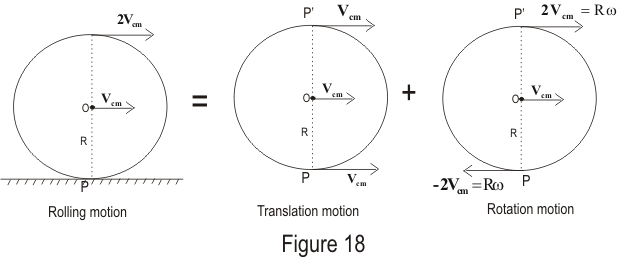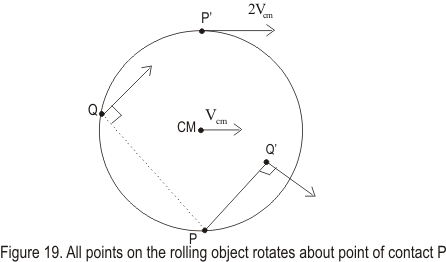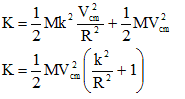# Rolling Motion

## Rolling Motion

• Let us now understand the motion of rolling body. For this consider a body with circular symmetry for example cylinder, wheel, disc , sphere etc.
• When such a body rolls on a plane surface , the motion of such a body is a combination of translational motion and rotational motion as shown below in the figure.• At any instant the axis normal to the digram through the point of contact P is the axis of rotation. If the speed of the centre of mass relative to an observer fixed on the surface is Vcm then the instantaneous angular speed about an axis through P would be
ω=Vcm/R
where R is the radius of the body.
• To explain this consider the figure given belowAt any instant different particles of the body have different linear speeds. The point P is at rest Vcm=0 instantaneously , the centre of mass has speed Vcm=Rω and the highest point on the circumference p' has speed Vcm=2Rω relative to point P.
• Now again consider the first figure the top of the cylinder has linear speed Vcm+ Rω=Vcm+Vcm=2Vcm, which is greater than the linear speed of any other point on the cylinder. We thus note that the Center of mass moves with linear speed Vcm while the contact point between the surface and rolling object has a linear speed of zero.

• Therefore at that instant all particles of the rigid body are moving with the same angular speed ω about the axis through P and the motion of the body is equivalent to pure rotational motion.

## Kinetic Energy of rolling bodies

• Now let us calculate the kinetic energy of the rolling bodes
• From above paragraph, it is clear that at any instant all particles of the rigid body are moving with the same angular speed ω about the axis through P and the motion of the body is equivalent to pure rotational motion
• Thus total kinetic energy is
K=½(IPω2)
where IP is the moment of inertia of the rigid body about point P.
• From parallel axis theorem
IP=Icm+MR2
where Icm is the moment of inertia of the body of mass M about parallel axis through point O.
Therefore
K=½(Icmω2)+½(MR2ω2)=½(Icmω2)+½(M(Vcm)2)
here the first term represents the rotational kinetic energy of the cylinder about its center of mass, and the second term represents the kinetic energy the cylinder would have if it were just translating through space without rotating. Thus, we can say that the total kinetic energy of a rolling object is the sum of the rotational kinetic energy about the center of mass and the translational kinetic energy of the center of mass.
• If k is the radius of gyration of the body about a parallel axis through O then I=Mk2 and total kinetic energy would then be,Question 1.A cylinder rolls up the incline plane reaches some height and then roll down without slipping through out this section.The direction of the frictional force acting on the cylinder are
a. Up the incline while ascending and down the incline while descending
b.Up the incline while ascending and descending
c. down the incline while ascending and up the incline while descending
d.down the incline while ascending and descending
Solution 3:
Imagine the cylinder to be moving on a frictionless surface.In both the cases the acceleration of the CM of the cylinder is gsinθ.This is also the acceleration of the point of contact
of the cylinder and the inclined plane..Also no torque (about the center of the cylinder) is acting on the cylinder since we assumed the surface to be a frictionless and the forces
acting on the cylinder is mg and N which passes through the center of the cylinder.Therefore the net movement of the point of contact in both the cases is in downward direction
Therefore frictional force will act in upward direction in both the cases

Question 2.A uniform sold sphere rolls on the horizontal surface at 20 m/s.it then rolls up the incline of 30.If friction losses are negligible what will be the value of h where sphere stops on the incline
a. 28.6 m
b 30 m
c. 28 m
d. none of these
Solution
Let h be the height
The rotational and translational KE of the ball at the bottom will be changed to Gravitational energy when the sphere stops .
We therefore writes

(1/2)Mv2+(1/2)Iω2=Mgh

For a solid sphere I=(2/5)Mr2 and also ω=v/r

So (1/2)Mv2+ (1/2)(2/5)Mr2(v/r)2=Mgh
or
v2/2 +v2/5=gh

or h=28.6 m

Question 3. A cylinder of Mass M and radius R rolls down a incline plane of inclination θ.Find the linear acceleration of the cylinder
a. (2/3)gsinθ
b.(2/3)gcosθ
c gsinθ
d none of these
Solution
Net force on the cylinder
Fnet=mgsinθ -f
or ma=mgsinθ -f
Where f is the frictional force
Now τ=fXR=Iα
Now in case of pure rolling we know that
a=αR => α=a/R

So f=Ia/R2

From 1 and 2
a=mgsinθ /[m+(I/R2)]

Now I=mR2/2

So a=(2/3)gsinθ